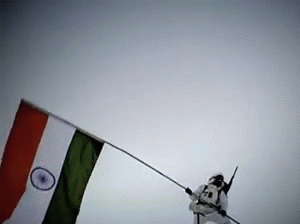### DICE Questions Simple and Shortcut tricks

DICE Questions Simple and Shortcut tricks

Hello Readers, looking for DICE Questions Simple tricks.Today we will be learning regarding one of the important topic for verbal reasoning - DICE. Its generally asked in many exams and has important weightage for reasoning exam paper. So candidates need to focus on such questions too. This questions are most easy easy if understood the working of dice with below given rules. You will able to solve the questions quickly and easily with accurate results. So candidates go through the article below and checkout the way to solve the questions quickly. For any questions feel free to ask questions in below comments.

### DICE Questions Simple and Shortcut tricks

Dice is a cube. In cube there are 6 faces. Some important points are given below:There are 6 faces in the cube - ABCG, GCDE, DEFH, BCDH, AGEF and ABHF.

Always four faces are adjacent to one face.
Opposite of ABCG is DEFH and so on.
CDEG is the upper face of the cube.

ABHF is the bottom of the cube.

Certain rules will help of to solve dice question easily and quickly.

Rule No. 1:

Two opposite faces cannot be adjacent to one another.

Example:

Two different positions of a dice are shown below. Which number will appear on the face opposite to the face with number 4?

Solution:

Faces with four numbers 6, 2, 5 and 3 are adjacent of to the face with No. 4.
Hence the faces with no. 6, 2, 5 and 3 cannot be opposite to the face with no. 4.
Therefore the remaining face with no.1 will be the opposite of the face with no. 4.

Rule No. 2:

If two different positions of a dice are shown and one of the two common faces is in the same position then of the remaining faces will be opposite to each other.

Example:

Two different positions of a dice are shown below.Here in both shown positions two faces 5 and 3 are common.
The remaining faces are 2 and 4.
Hence the number on the face opposite to the face with number 2 is 4.

Rule No. 3:

If in two different positions of dice, the position of a common face be the same, then each of the opposite faces of the remaining faces will be in the same position.

Example:

Here in both positions of common (3) is same.
Therefore, opposite of 5 is 6 and opposite of 4 is 2.

Rule No. 4:

If in two different positions of a dice, the position of the common face be not the same, then opposite face of the common face will be that which is not shown on any face in these two positions. Besides, the opposite faces of the remaining faces will not be the same.

Example:Here in two positions of a dice the face with number 1 is not in the same position.
The face with number 6 is not shown.
Hence the face opposite to the face with number 1 is 6.
Besides the opposite face of 3 will be the face with number 2 and opposite face to face 5 will be the face with number 1.

So this was all about DICE Questions Simple and Shortcut tricks. Hope now you know how to solve dice questions quickly and accurately. For any help please feel free to ask below in comments regarding DICE Questions Simple and Shortcut tricks.

## .